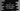# Find 'sin' 'cos' and 'tan' values of a 'degree' in C### Find ‘sin’ ‘cos’ and ‘tan’ values of a ‘degree’ in C :

We have one standard math.h library function in C that helps to find the ‘sin’, ‘cos’ and ‘tan’ value of a degree. In this tutorial, we will learn how to calculate these values from a user input degree value. Let’s take a look at how to do this:

### C program :

``````#include <stdio.h>
#include <math.h>

int main()
{
double degree;

printf("Enter your angle value in degree : ");
scanf("%lf", &degree);

double radian = degree * (M_PI / 180);

printf("sin value is %lf \n", sin(radian));
printf("cos value is %lf \n", cos(radian));
printf("tan value is %lf \n", tan(radian));
return 0;
}``````

### Explanation :

1. To solve this problem, we need to import math.h header function.
2. All of these methods take one double value as the argument and returns the result as double.
3. The argument should be in radian, so if the user input is in degree, we need to convert it to radian by multiplying the value PI/180. MI_PI is defined in math.h and it holds the value of PI.
4. After converting it to radian, we can calculate and print out the sin, cos and tan values.
5. To make the conversion, we can use sin(), cos() and tan() methods.

### Sample Output :

``````Enter your angle value in degree : 60
sin value is 0.866025
cos value is 0.500000
tan value is 1.732051

sin value is 0.500000
cos value is 0.866025
tan value is 0.577350

Enter your angle value in degree : 45
sin value is 0.707107
cos value is 0.707107
tan value is 1.000000``````